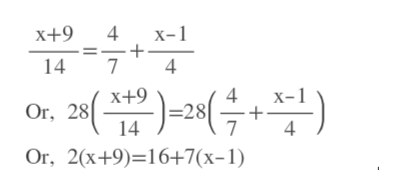# X+9 divided by 14= 4 divided by 7 +x-1 divided by 4

Question
1 views

X+9 divided by 14= 4 divided by 7 +x-1 divided by 4

check_circle

star
star
star
star
star
1 Rating
Step 1

First find LCm of the denominators.

LCM of 14,7,4= 28

We multiply both sides by 28.help_outlineImage Transcriptioncloseх+9 х-1 7 14 х+9 4 -28 7 x- Or, 28 14 4 Or, 2(x+9)-16+7(х-1) fullscreen
Step 2

Distribute 2 and 7 and then simplify both sides.

Step 3

Subtract 7x from bot...

### Want to see the full answer?

See Solution

#### Want to see this answer and more?

Solutions are written by subject experts who are available 24/7. Questions are typically answered within 1 hour.*

See Solution
*Response times may vary by subject and question.
Tagged in

### Equations and In-equations